# Paired Z-Test of Proportions

Where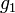$g_1$ and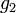$g_2$ are the two proportions and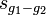$s_{g_1 - g_2}$ is an estimate of the standard error of the difference between the proportions: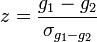$z=\frac{g_1-g_2}{\sigma_{g_1-g_2}}$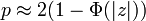$p \approx 2(1-\Phi(|z|))$ if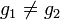$g_1 \ne g_2$ and NaN otherwise,$s_{g_1 - g_2}$ is computed as the Standard Error of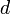$d$,
the value for the$i$th observation is computed as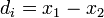$d_i = x_1 - x_2$,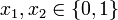$x_1,x_2 \in {\{0,1\}}$ are the observed values on the two variables.